### Beace Blog

Written by Beace Lee who lives and works in China building useful things. You should follow him on Twitter

# JavaScript-Math.random()

February 22, 2017

# Math.random()

## 伪随机

`Math.random()`返回的是一个左闭右开 `[0,1)`的伪随机浮点数。之所以称作伪随机，是因为整个过程看起来像是最随机的，如下图，我们也看到了随机数的分布。但是实际上并不是。不是的原因是——我们是借助一个固定的方法来获取数据。并且数据最终是可控的，因为我们固定了它的区间。

``2.3/10 //0.22999999999999998``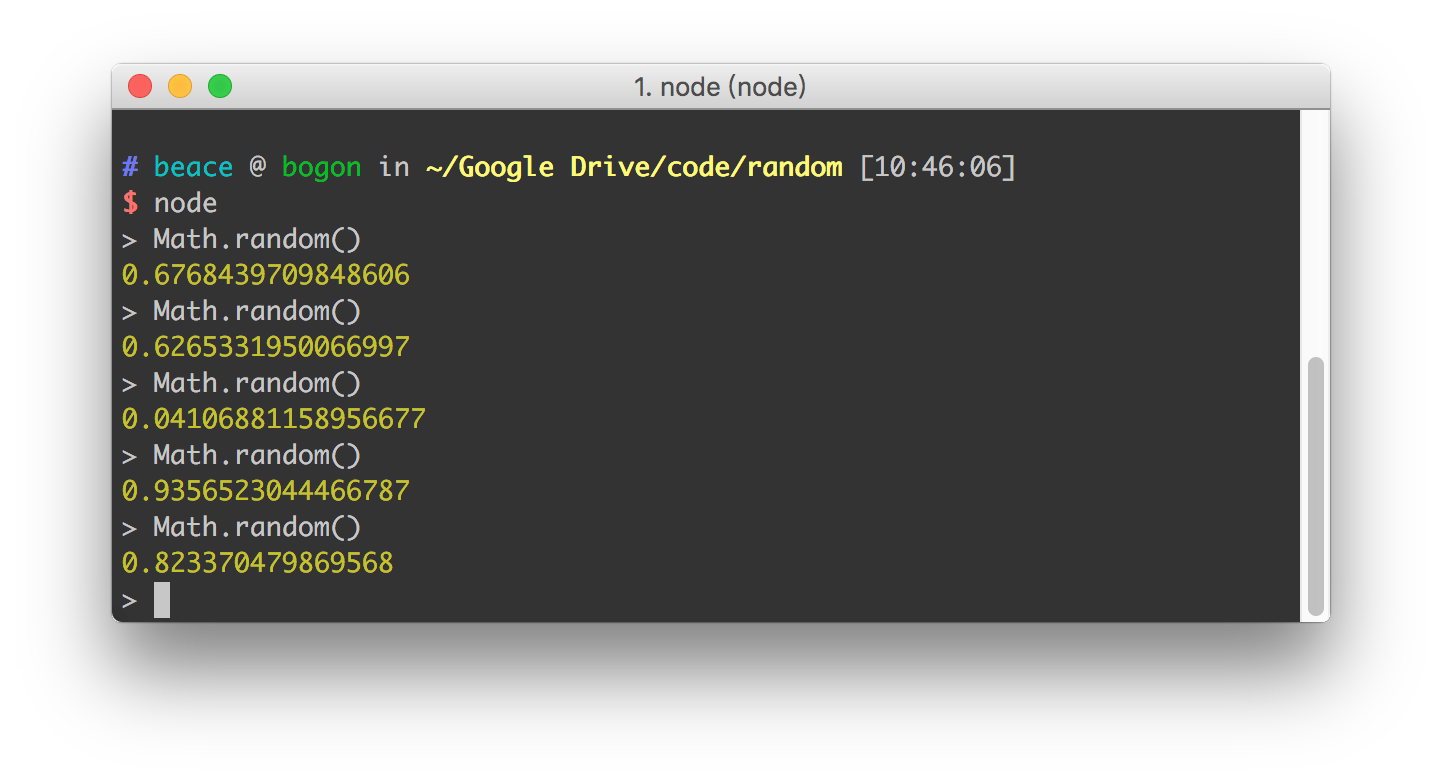## 随机数

• `[0, n)`随机数选取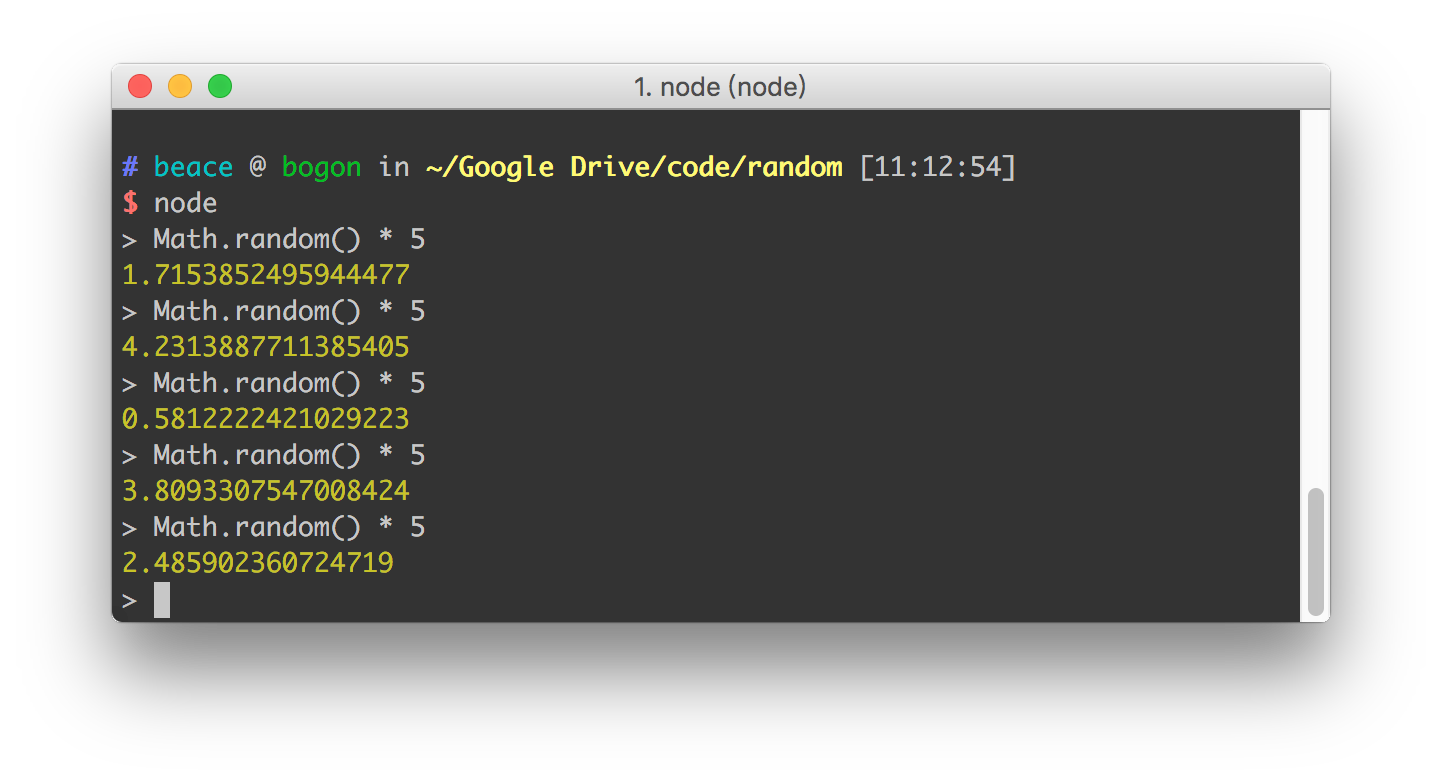• `[m, n]`随机数选取

``````//m n 为整形
function getM2NRandom(m, n) {
return Math.random() * (n - m) + m;
}``````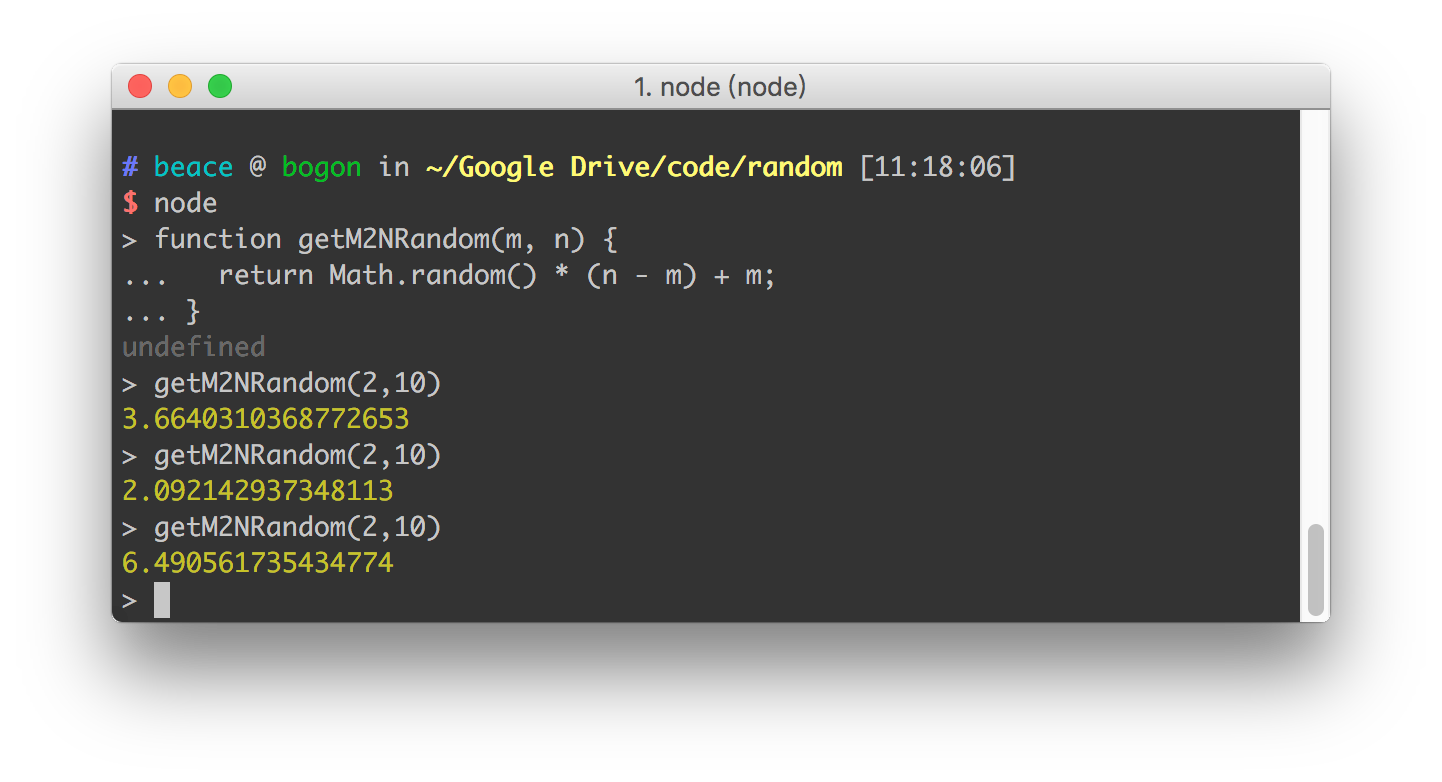## 取整数

• `Math.ceil()`

``````Math.ceil(2.44444) // 3
Math.ceil(2.99944) // 3
Math.ceil(2) // 2``````
• `Math.floor()`

``````Math.floor(2.44444) // 2
Math.floor(2.99944) // 2
Math.floor(2) //2``````

## 随机整数

• `[0, n)随机取整`

``````function getIntRandom(n) {
console.log(Math.ceil(Math.random() * n));
console.log(Math.floor(Math.random() * n));
}
getIntRandom(10);``````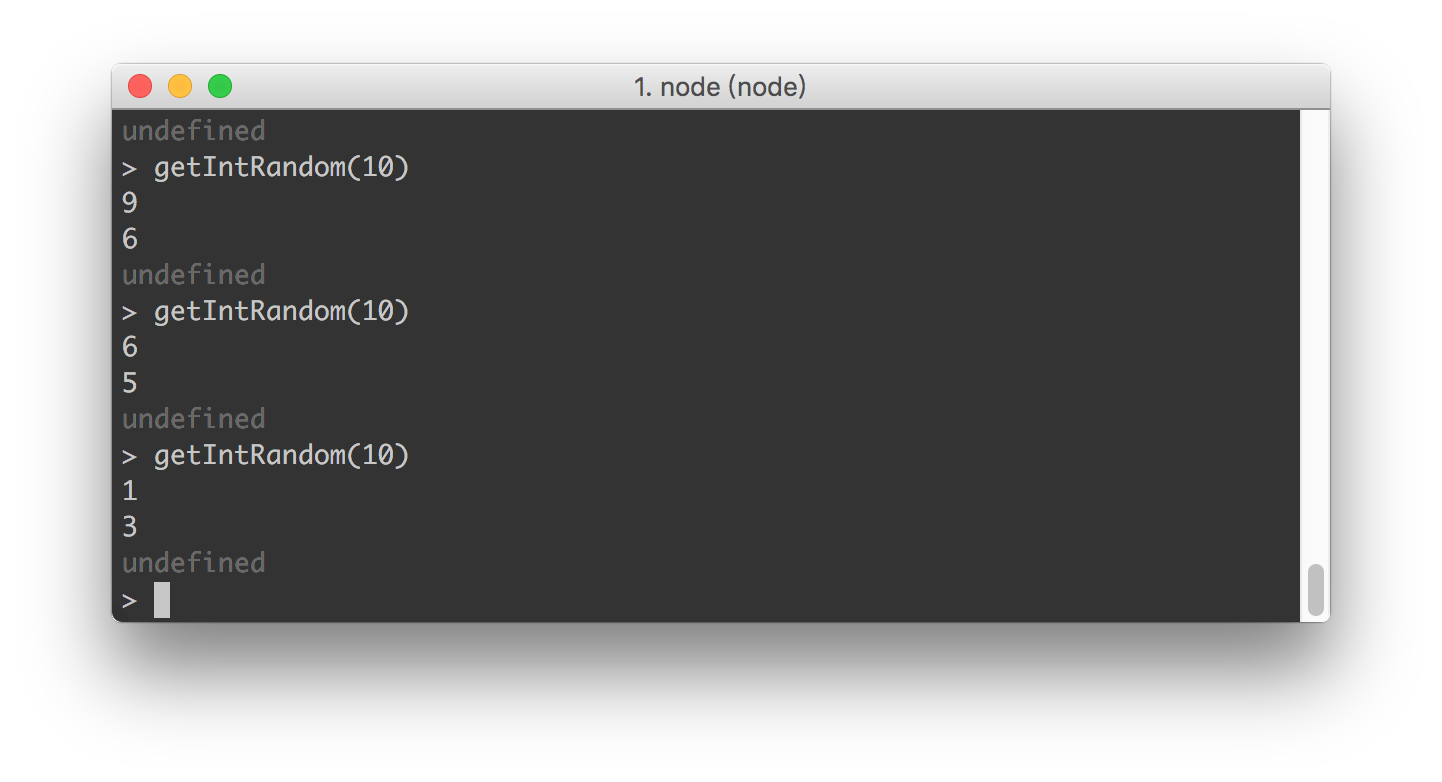• `[m, n]`随机取整
``````function getIntM2NRandom(m , n) {
return Math.floor(Math.random() * (n - m + 1) + n)
}
getIntM2NRandom(2, 5);``````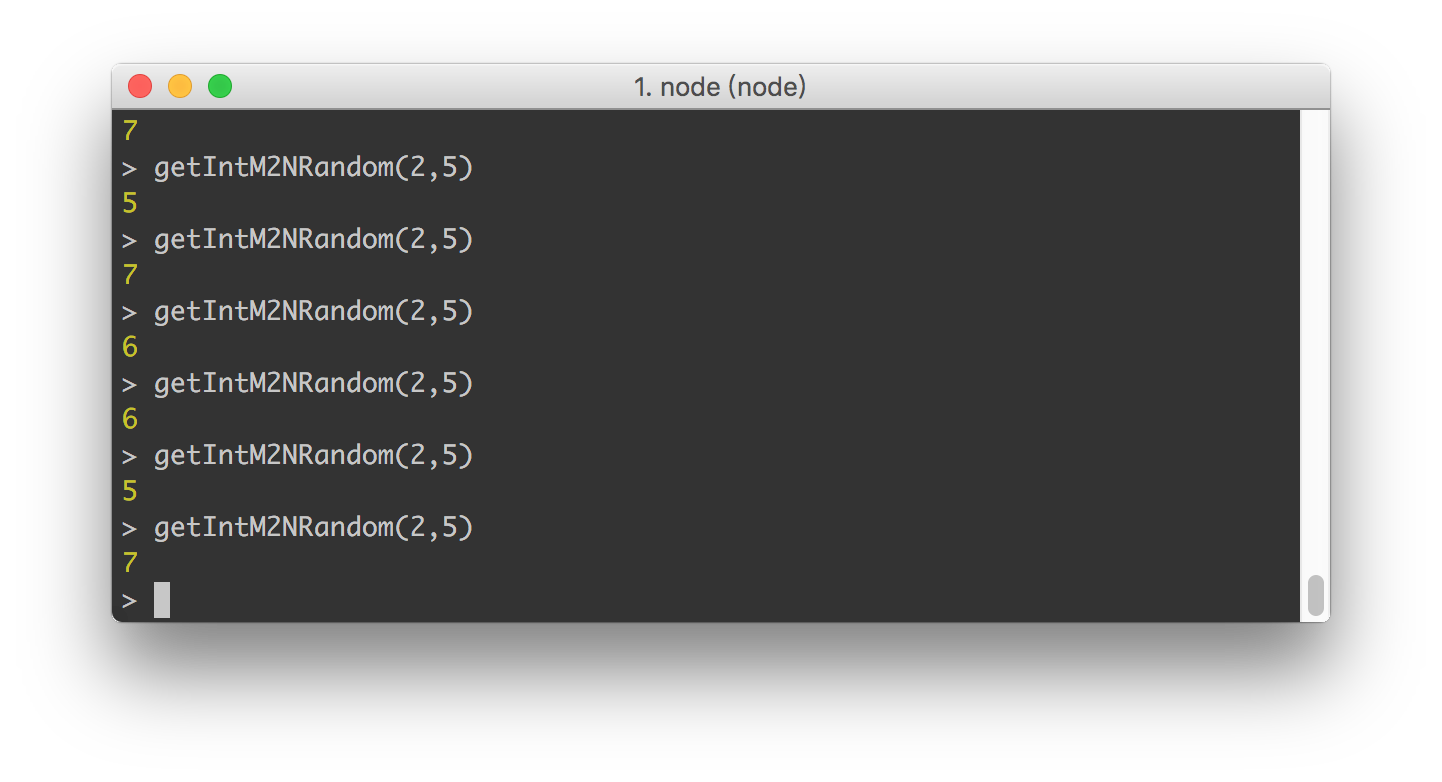## 实际应用

``````//初始化一个 1~62 的数组
const cards = Array(62).fill().map((_,i)=>i+1);
// 一次抽取 n 个，默认一次 1 个
function draw(n = 1){
var ret = [];
for(var i = 0; i < n; i++){
let idx = Math.floor(cards.length * Math.random());
ret.push(...cards.splice(idx, 1));
}
return ret;
}
//抽取一次，10个中奖者
console.log(draw(10));``````